# 4.4 Graphs of logarithmic functions  (Page 8/8)

 Page 8 / 8

## Verbal

The inverse of every logarithmic function is an exponential function and vice-versa. What does this tell us about the relationship between the coordinates of the points on the graphs of each?

Since the functions are inverses, their graphs are mirror images about the line $\text{\hspace{0.17em}}y=x.\text{\hspace{0.17em}}$ So for every point $\text{\hspace{0.17em}}\left(a,b\right)\text{\hspace{0.17em}}$ on the graph of a logarithmic function, there is a corresponding point $\text{\hspace{0.17em}}\left(b,a\right)\text{\hspace{0.17em}}$ on the graph of its inverse exponential function.

What type(s) of translation(s), if any, affect the range of a logarithmic function?

What type(s) of translation(s), if any, affect the domain of a logarithmic function?

Shifting the function right or left and reflecting the function about the y-axis will affect its domain.

Consider the general logarithmic function $\text{\hspace{0.17em}}f\left(x\right)={\mathrm{log}}_{b}\left(x\right).\text{\hspace{0.17em}}$ Why can’t $\text{\hspace{0.17em}}x\text{\hspace{0.17em}}$ be zero?

Does the graph of a general logarithmic function have a horizontal asymptote? Explain.

No. A horizontal asymptote would suggest a limit on the range, and the range of any logarithmic function in general form is all real numbers.

## Algebraic

For the following exercises, state the domain and range of the function.

$f\left(x\right)={\mathrm{log}}_{3}\left(x+4\right)$

$h\left(x\right)=\mathrm{ln}\left(\frac{1}{2}-x\right)$

Domain: $\text{\hspace{0.17em}}\left(-\infty ,\frac{1}{2}\right);\text{\hspace{0.17em}}$ Range: $\text{\hspace{0.17em}}\left(-\infty ,\infty \right)$

$g\left(x\right)={\mathrm{log}}_{5}\left(2x+9\right)-2$

$h\left(x\right)=\mathrm{ln}\left(4x+17\right)-5$

Domain: $\text{\hspace{0.17em}}\left(-\frac{17}{4},\infty \right);\text{\hspace{0.17em}}$ Range: $\text{\hspace{0.17em}}\left(-\infty ,\infty \right)$

$f\left(x\right)={\mathrm{log}}_{2}\left(12-3x\right)-3$

For the following exercises, state the domain and the vertical asymptote of the function.

$\text{\hspace{0.17em}}f\left(x\right)={\mathrm{log}}_{b}\left(x-5\right)$

Domain: $\text{\hspace{0.17em}}\left(5,\infty \right);\text{\hspace{0.17em}}$ Vertical asymptote: $\text{\hspace{0.17em}}x=5$

$\text{\hspace{0.17em}}g\left(x\right)=\mathrm{ln}\left(3-x\right)$

$\text{\hspace{0.17em}}f\left(x\right)=\mathrm{log}\left(3x+1\right)$

Domain: $\text{\hspace{0.17em}}\left(-\frac{1}{3},\infty \right);\text{\hspace{0.17em}}$ Vertical asymptote: $\text{\hspace{0.17em}}x=-\frac{1}{3}$

$\text{\hspace{0.17em}}f\left(x\right)=3\mathrm{log}\left(-x\right)+2$

$\text{\hspace{0.17em}}g\left(x\right)=-\mathrm{ln}\left(3x+9\right)-7$

Domain: $\text{\hspace{0.17em}}\left(-3,\infty \right);\text{\hspace{0.17em}}$ Vertical asymptote: $\text{\hspace{0.17em}}x=-3$

For the following exercises, state the domain, vertical asymptote, and end behavior of the function.

$f\left(x\right)=\mathrm{ln}\left(2-x\right)$

$f\left(x\right)=\mathrm{log}\left(x-\frac{3}{7}\right)$

Domain: $\left(\frac{3}{7},\infty \right)$ ;
Vertical asymptote: $x=\frac{3}{7}$ ; End behavior: as $x\to {\left(\frac{3}{7}\right)}^{+},f\left(x\right)\to -\infty$ and as $x\to \infty ,f\left(x\right)\to \infty$

$h\left(x\right)=-\mathrm{log}\left(3x-4\right)+3$

$g\left(x\right)=\mathrm{ln}\left(2x+6\right)-5$

Domain: $\left(-3,\infty \right)$ ; Vertical asymptote: $x=-3$ ;
End behavior: as $x\to -{3}^{+}$ , $f\left(x\right)\to -\infty$ and as $x\to \infty$ , $f\left(x\right)\to \infty$

$f\left(x\right)={\mathrm{log}}_{3}\left(15-5x\right)+6$

For the following exercises, state the domain, range, and x - and y -intercepts, if they exist. If they do not exist, write DNE.

$h\left(x\right)={\mathrm{log}}_{4}\left(x-1\right)+1$

Domain: $\text{\hspace{0.17em}}\left(1,\infty \right);\text{\hspace{0.17em}}$ Range: $\text{\hspace{0.17em}}\left(-\infty ,\infty \right);\text{\hspace{0.17em}}$ Vertical asymptote: $\text{\hspace{0.17em}}x=1;\text{\hspace{0.17em}}$ x -intercept: $\text{\hspace{0.17em}}\left(\frac{5}{4},0\right);\text{\hspace{0.17em}}$ y -intercept: DNE

$f\left(x\right)=\mathrm{log}\left(5x+10\right)+3$

$g\left(x\right)=\mathrm{ln}\left(-x\right)-2$

Domain: $\text{\hspace{0.17em}}\left(-\infty ,0\right);\text{\hspace{0.17em}}$ Range: $\text{\hspace{0.17em}}\left(-\infty ,\infty \right);\text{\hspace{0.17em}}$ Vertical asymptote: $\text{\hspace{0.17em}}x=0;\text{\hspace{0.17em}}$ x -intercept: $\text{\hspace{0.17em}}\left(-{e}^{2},0\right);\text{\hspace{0.17em}}$ y -intercept: DNE

$f\left(x\right)={\mathrm{log}}_{2}\left(x+2\right)-5$

$h\left(x\right)=3\mathrm{ln}\left(x\right)-9$

Domain: $\text{\hspace{0.17em}}\left(0,\infty \right);\text{\hspace{0.17em}}$ Range: $\text{\hspace{0.17em}}\left(-\infty ,\infty \right);\text{\hspace{0.17em}}$ Vertical asymptote: $\text{\hspace{0.17em}}x=0;\text{\hspace{0.17em}}$ x -intercept: $\text{\hspace{0.17em}}\left({e}^{3},0\right);\text{\hspace{0.17em}}$ y -intercept: DNE

## Graphical

For the following exercises, match each function in [link] with the letter corresponding to its graph.

$d\left(x\right)=\mathrm{log}\left(x\right)$

$f\left(x\right)=\mathrm{ln}\left(x\right)$

B

$g\left(x\right)={\mathrm{log}}_{2}\left(x\right)$

$h\left(x\right)={\mathrm{log}}_{5}\left(x\right)$

C

$j\left(x\right)={\mathrm{log}}_{25}\left(x\right)$

For the following exercises, match each function in [link] with the letter corresponding to its graph.

$f\left(x\right)={\mathrm{log}}_{\frac{1}{3}}\left(x\right)$

B

$g\left(x\right)={\mathrm{log}}_{2}\left(x\right)$

$h\left(x\right)={\mathrm{log}}_{\frac{3}{4}}\left(x\right)$

C

For the following exercises, sketch the graphs of each pair of functions on the same axis.

$f\left(x\right)=\mathrm{log}\left(x\right)\text{\hspace{0.17em}}$ and $\text{\hspace{0.17em}}g\left(x\right)={10}^{x}$

$f\left(x\right)=\mathrm{log}\left(x\right)\text{\hspace{0.17em}}$ and $\text{\hspace{0.17em}}g\left(x\right)={\mathrm{log}}_{\frac{1}{2}}\left(x\right)$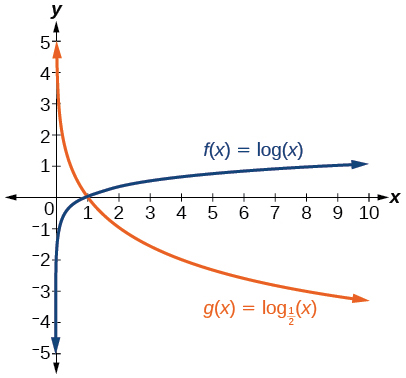$f\left(x\right)={\mathrm{log}}_{4}\left(x\right)\text{\hspace{0.17em}}$ and $\text{\hspace{0.17em}}g\left(x\right)=\mathrm{ln}\left(x\right)$

$f\left(x\right)={e}^{x}\text{\hspace{0.17em}}$ and $\text{\hspace{0.17em}}g\left(x\right)=\mathrm{ln}\left(x\right)$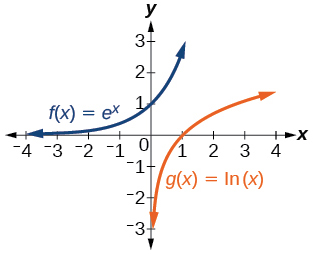For the following exercises, match each function in [link] with the letter corresponding to its graph.

$f\left(x\right)={\mathrm{log}}_{4}\left(-x+2\right)$

$g\left(x\right)=-{\mathrm{log}}_{4}\left(x+2\right)$

C

$h\left(x\right)={\mathrm{log}}_{4}\left(x+2\right)$

For the following exercises, sketch the graph of the indicated function.

$\text{\hspace{0.17em}}f\left(x\right)={\mathrm{log}}_{2}\left(x+2\right)$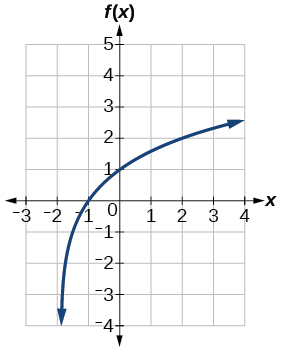$\text{\hspace{0.17em}}f\left(x\right)=2\mathrm{log}\left(x\right)$

$\text{\hspace{0.17em}}f\left(x\right)=\mathrm{ln}\left(-x\right)$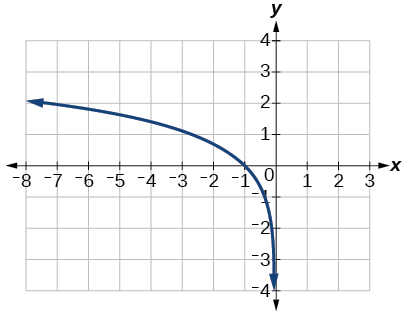$g\left(x\right)=\mathrm{log}\left(4x+16\right)+4$

$g\left(x\right)=\mathrm{log}\left(6-3x\right)+1$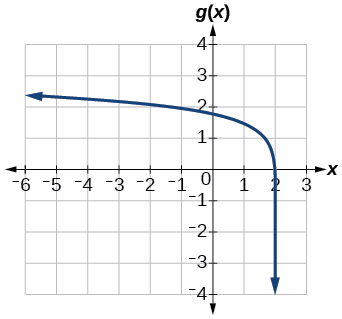$h\left(x\right)=-\frac{1}{2}\mathrm{ln}\left(x+1\right)-3$

For the following exercises, write a logarithmic equation corresponding to the graph shown.

Use $\text{\hspace{0.17em}}y={\mathrm{log}}_{2}\left(x\right)\text{\hspace{0.17em}}$ as the parent function.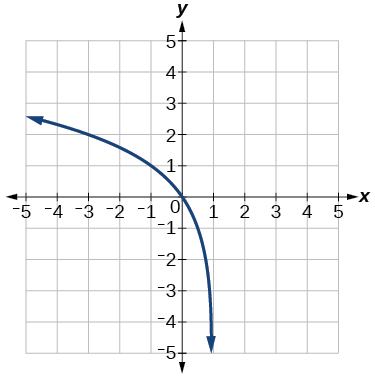$\text{\hspace{0.17em}}f\left(x\right)={\mathrm{log}}_{2}\left(-\left(x-1\right)\right)$

Use $\text{\hspace{0.17em}}f\left(x\right)={\mathrm{log}}_{3}\left(x\right)\text{\hspace{0.17em}}$ as the parent function.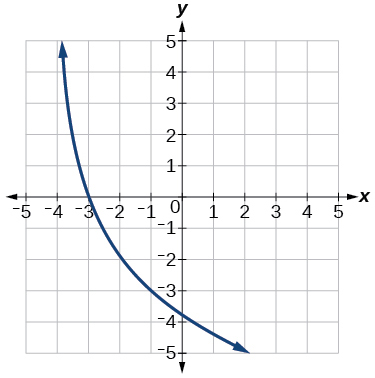Use $\text{\hspace{0.17em}}f\left(x\right)={\mathrm{log}}_{4}\left(x\right)\text{\hspace{0.17em}}$ as the parent function.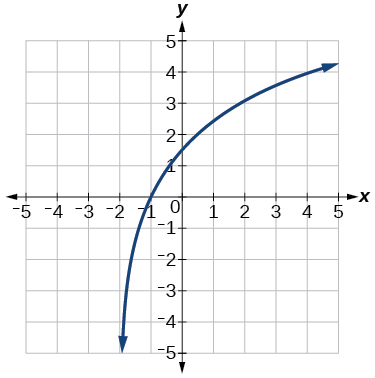$f\left(x\right)=3{\mathrm{log}}_{4}\left(x+2\right)$

Use $\text{\hspace{0.17em}}f\left(x\right)={\mathrm{log}}_{5}\left(x\right)\text{\hspace{0.17em}}$ as the parent function.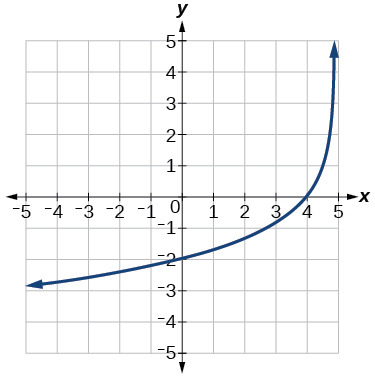## Technology

For the following exercises, use a graphing calculator to find approximate solutions to each equation.

$\mathrm{log}\left(x-1\right)+2=\mathrm{ln}\left(x-1\right)+2$

$x=2$

$\mathrm{log}\left(2x-3\right)+2=-\mathrm{log}\left(2x-3\right)+5$

$\mathrm{ln}\left(x-2\right)=-\mathrm{ln}\left(x+1\right)$

$x\approx \text{2}\text{.303}$

$2\mathrm{ln}\left(5x+1\right)=\frac{1}{2}\mathrm{ln}\left(-5x\right)+1$

$\frac{1}{3}\mathrm{log}\left(1-x\right)=\mathrm{log}\left(x+1\right)+\frac{1}{3}$

$x\approx -0.472$

## Extensions

Let $\text{\hspace{0.17em}}b\text{\hspace{0.17em}}$ be any positive real number such that $\text{\hspace{0.17em}}b\ne 1.\text{\hspace{0.17em}}$ What must $\text{\hspace{0.17em}}{\mathrm{log}}_{b}1\text{\hspace{0.17em}}$ be equal to? Verify the result.

Explore and discuss the graphs of $\text{\hspace{0.17em}}f\left(x\right)={\mathrm{log}}_{\frac{1}{2}}\left(x\right)\text{\hspace{0.17em}}$ and $\text{\hspace{0.17em}}g\left(x\right)=-{\mathrm{log}}_{2}\left(x\right).\text{\hspace{0.17em}}$ Make a conjecture based on the result.

The graphs of $\text{\hspace{0.17em}}f\left(x\right)={\mathrm{log}}_{\frac{1}{2}}\left(x\right)\text{\hspace{0.17em}}$ and $\text{\hspace{0.17em}}g\left(x\right)=-{\mathrm{log}}_{2}\left(x\right)\text{\hspace{0.17em}}$ appear to be the same; Conjecture: for any positive base $\text{\hspace{0.17em}}b\ne 1,$ $\text{\hspace{0.17em}}{\mathrm{log}}_{b}\left(x\right)=-{\mathrm{log}}_{\frac{1}{b}}\left(x\right).$

Prove the conjecture made in the previous exercise.

What is the domain of the function $\text{\hspace{0.17em}}f\left(x\right)=\mathrm{ln}\left(\frac{x+2}{x-4}\right)?\text{\hspace{0.17em}}$ Discuss the result.

Recall that the argument of a logarithmic function must be positive, so we determine where $\text{\hspace{0.17em}}\frac{x+2}{x-4}>0\text{\hspace{0.17em}}$ . From the graph of the function $\text{\hspace{0.17em}}f\left(x\right)=\frac{x+2}{x-4},$ note that the graph lies above the x -axis on the interval $\text{\hspace{0.17em}}\left(-\infty ,-2\right)\text{\hspace{0.17em}}$ and again to the right of the vertical asymptote, that is $\text{\hspace{0.17em}}\left(4,\infty \right).\text{\hspace{0.17em}}$ Therefore, the domain is $\text{\hspace{0.17em}}\left(-\infty ,-2\right)\cup \left(4,\infty \right).$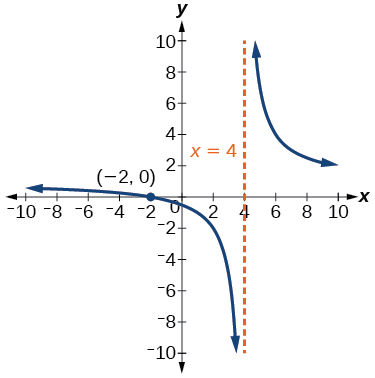Use properties of exponents to find the x -intercepts of the function $\text{\hspace{0.17em}}f\left(x\right)=\mathrm{log}\left({x}^{2}+4x+4\right)\text{\hspace{0.17em}}$ algebraically. Show the steps for solving, and then verify the result by graphing the function.

how fast can i understand functions without much difficulty
what is set?
a colony of bacteria is growing exponentially doubling in size every 100 minutes. how much minutes will it take for the colony of bacteria to triple in size
I got 300 minutes. is it right?
Patience
no. should be about 150 minutes.
Jason
It should be 158.5 minutes.
Mr
ok, thanks
Patience
100•3=300 300=50•2^x 6=2^x x=log_2(6) =2.5849625 so, 300=50•2^2.5849625 and, so, the # of bacteria will double every (100•2.5849625) = 258.49625 minutes
Thomas
what is the importance knowing the graph of circular functions?
can get some help basic precalculus
What do you need help with?
Andrew
how to convert general to standard form with not perfect trinomial
can get some help inverse function
ismail
Rectangle coordinate
how to find for x
it depends on the equation
Robert
yeah, it does. why do we attempt to gain all of them one side or the other?
Melissa
whats a domain
The domain of a function is the set of all input on which the function is defined. For example all real numbers are the Domain of any Polynomial function.
Spiro
Spiro; thanks for putting it out there like that, 😁
Melissa
foci (–7,–17) and (–7,17), the absolute value of the differenceof the distances of any point from the foci is 24.
difference between calculus and pre calculus?
give me an example of a problem so that I can practice answering
x³+y³+z³=42
Robert
dont forget the cube in each variable ;)
Robert
of she solves that, well ... then she has a lot of computational force under her command ....
Walter
what is a function?
I want to learn about the law of exponent
explain this

#### Get Jobilize Job Search Mobile App in your pocket Now!ByByByBy Dakota BocanBy OpenStaxBy RhodesBy Sandy YamaneBy OpenStaxBy Steve GibbsBy IES PortalBy Brenna Fike